You are using an outdated browser. Please upgrade your browser to improve your experience.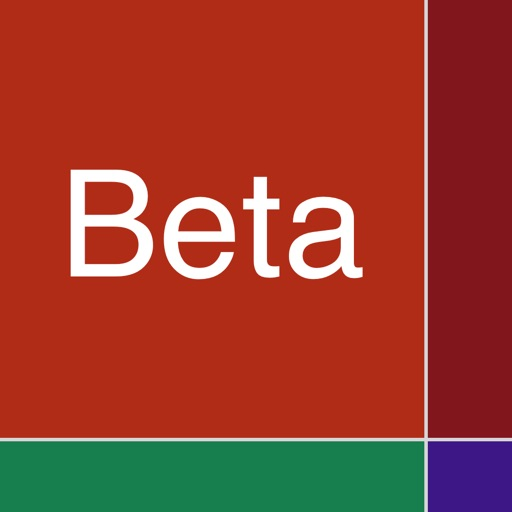## In probability theory and statistics, the beta-binomial distribution is a family of discrete probability distributions on a finite support of non-negative integers arising when the probability of success in each of a fixed or known number of Bernoulli trials is either unknown or random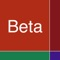# Beta-binomial Distribution

by Donald Schaefer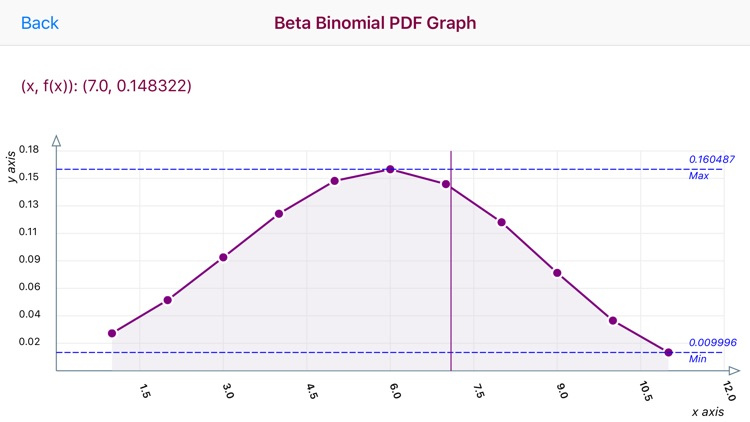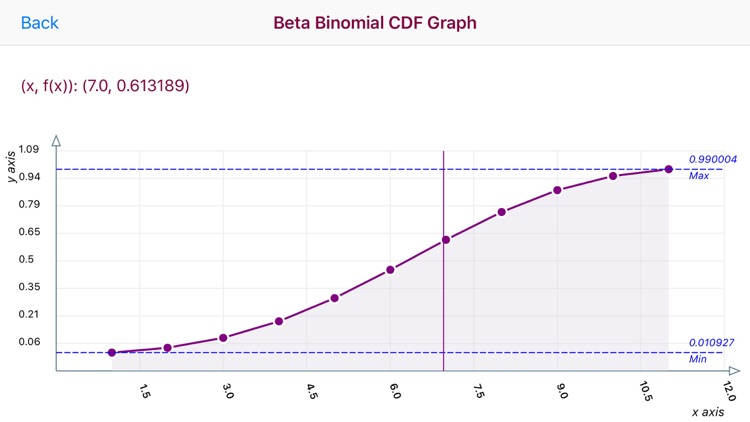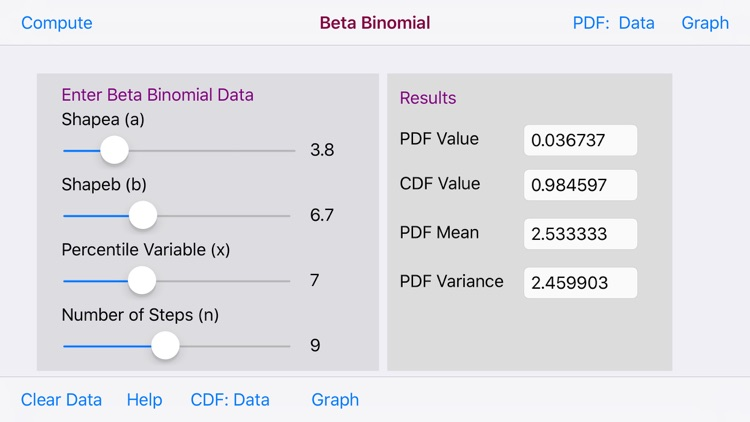### What is it about?

In probability theory and statistics, the beta-binomial distribution is a family of discrete probability distributions on a finite support of non-negative integers arising when the probability of success in each of a fixed or known number of Bernoulli trials is either unknown or random. The beta-binomial distribution is the binomial distribution in which the probability of success at each trial is not fixed but random and follows the beta distribution### App Details

Version
1.1
Rating
NA
Size
7Mb
Genre
Education Productivity
Last updated
January 22, 2019
Release date
November 22, 2016

### App Screenshots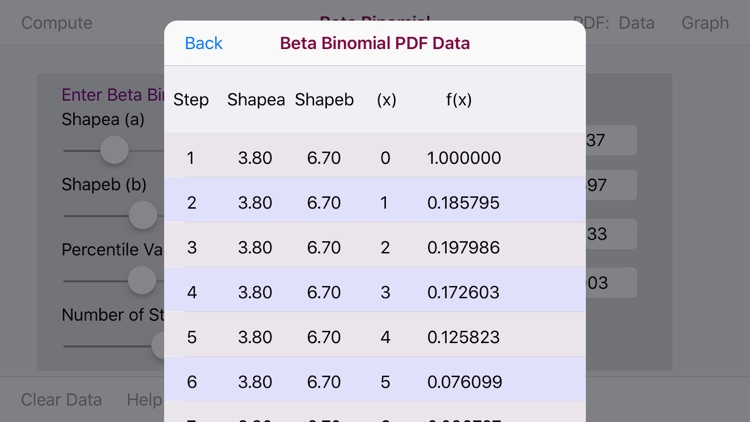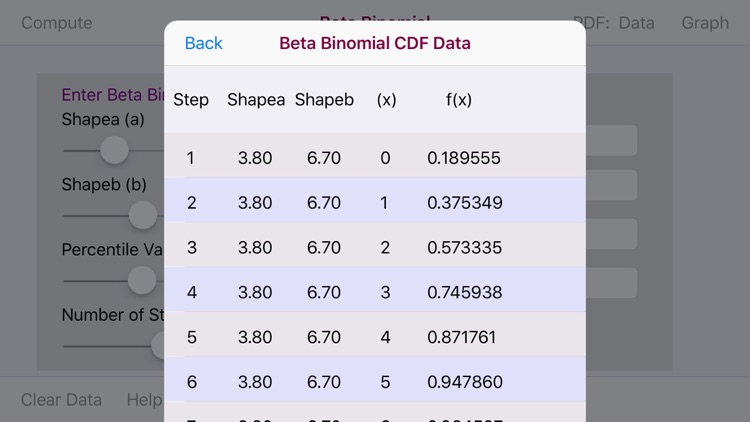### App Store Description

In probability theory and statistics, the beta-binomial distribution is a family of discrete probability distributions on a finite support of non-negative integers arising when the probability of success in each of a fixed or known number of Bernoulli trials is either unknown or random. The beta-binomial distribution is the binomial distribution in which the probability of success at each trial is not fixed but random and follows the beta distribution

For Data Entry the Beta Binomial Distribution app utilizes four Sliders for the Shape a, Shape b, Percentile Variable (x) and the number of steps parameters.

The Beta Binomial Distribution App displays PDF/CDF graphs and data table which displays (x/f(x)) data pairs. The Range of data displayed is the range of the x variable which is defined as 1.0 <= x <= the number of steps (n) variable.

The graphs are touch enabled.. Upon touching the graph a vertical line appears. Move the vertical line to the left or right to display the point (x/f(x)) data in the upper left of the graph.

The horizontal x-axis displays x values. The vertical y-axis plots a range of f(x) values.

Horizontal Max and Min dashed lines display the Maximum and Minimum f(x) values

Since the Beta Binomial Distribution is a Discrete Probability Distribution a dot (.) indicator is utilized to display each discrete data pair.

Disclaimer:
AppAdvice does not own this application and only provides images and links contained in the iTunes Search API, to help our users find the best apps to download. If you are the developer of this app and would like your information removed, please send a request to [email protected] and your information will be removed.